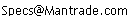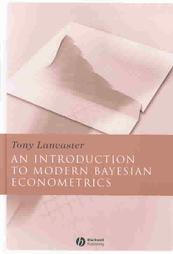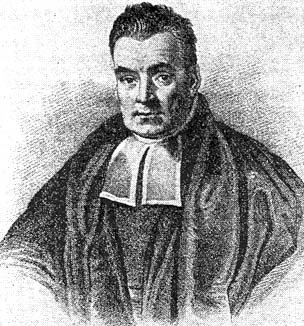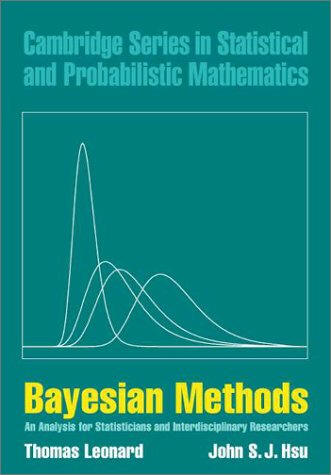Daily Speculations The Web Site of Victor Niederhoffer and Laurel KennerThe Chairman Victor Niederhoffer     About Victor Niederhoffer ©2006 All content on site protected by copyright

Write to us at:(address is not clickable)

28-Aug-2006
An Introduction to Modern Bayesian Econometrics, reviewed by Victor NiederhofferRight at the outset of Tony Lancaster's book, 'An Introduction to Modern Bayesian Econometrics' he states that it is probably better not to have any background in econometrics or statistics because the Bayesian approach is so different from the traditional ways. This book will change the way you think about decision making, prediction, and description in all economic matters, and includes computational techniques, an elaborate discussion of Bayes' theorem, and computer programs and code.

The basis for all the book is Bayes' theorem which the author emphasizes is the only way to revise beliefs based on numerical evidence. The basic theorem is as follows:

Suppose you have a theory that has different possibilities , say that the market is a 50-50 proposition or that it is biased to 60% up with a 10% per year drift. Let us call that your prior probability A with two states, A1 with a probability 0.5 and A2 with a probability 0.5. Next, consider what you would expect to find in evidence if your theories were true. That is event B. For example , if theory A1 were true you'd expect to find 4 rises in a row 0.06 of the time. But if theory A2 were true, you would expect to find 4 rises in a row 13% of the time. Now if you find four rises in a row, theory 2 becomes more likely by a factor 13/6.Originally the theory was 50-50 . but now the theories have an ex-post probabilities proportion to 0.5 x 13 and 0.5 x 0.06. So the revised the probabilities of A1 and A2 is that A1 is now 13/19 to be true, and A1 is now 6/19 to happen.

Formally, Bayes' theorem states that we start with a theory A and then revise it based on the evidence B according to the formula that the probability of A and B taken together is:

P (A) x P (B|A) = P(B) x P(A|B)

Alternately, P(A|B) = [P(B|A) / P(B)] x P(A)

The right most term in the numerator is the likelihood. The left most term is the revised posterior probability and the denominator on the right is the prior probability.

Bayes' theorem and all econometric decision making may then be formulated as a probability distribution for the various states of your hypothesis to find the likelihoods of various realizations of the data for each state of your hypothesis. Collect the data and then apply Bayes' theorem above to revise your probabilities. Then see how consistent your revised beliefs are with what you know about the situation, and how helpful it is for you to use it in the future, i.e. criticize and find out how useful your new model is through prediction.

The book contains numerous examples, numerous models, numerous extensions of this algorithm along with the computer code to perform such analysis. Topics treated include linear and non -linear regression models, multinomial models, autoregressive models, time series models, Markov Chain models, binary choice models, duration models, survey data and recursive equations. Extensive use of simulation is made throughout the book, and the book also includes a computer program to help make the models come to life. The book is somewhat technical, especially since all the results depend on computer modeling that yields output that is not clearly derivable by hand without, however, it will change the way you think about decision making, and will be an important adjunct in how you think in the future, regardless of the mastery of all the technical details contained. This book is highly recommended for a nice pencil and paper read.